Here we are providing Class 12 Chemistry Important Extra Questions and Answers Chapter 7 The p-Block Elements.  Class 12 Chemistry Important Questions are the best resource for students which helps in Class 12 board exams.

## Class 12 Chemistry Chapter 7 Important Extra Questions The p-Block Elements

### The p-Block Elements Important Extra Questions Very Short Answer Type

Question 1.
Which one of PCl4+ and PCl4 is not likely to exist and why? (CBSE Delhi 2012)
PCl4 is not likely to exist because lone pair of PCl3 can be donated to Cl+ and not to Cl.

Question 2.
Of PH3 and H2S which is more acidic and why? (CBSE Delhi 2012)
H2S is more acidic because of weak S-H bond. PH3 behaves as a Lewis base because of the presence of lone pair of electrons on P.

Question 3.
Which is a stronger reducing agent, SbH3 or BiH3 and why? (CBSE Al 2012)
BiH3 is a stronger reducing agent than SbH3. This is because BiH3 is less stable than SbH3 because of larger size of Bi than Sb.

Question 4.
What is the covalency of nitrogen in N2O5? (CBSE Delhi 2013)
Four.

Question 5.
Name two poisonous gases which can be prepared from chlorine gas. (CBSE AI 2013)

1. Phosgene (COCl2)
2. Mustard gas (ClCH2CH2SCH2CH2Cl)

Question 6.
What is the basicity of H3PO3 and why? (CBSE AI 2013)
H3PO3 contains two P-OH bonds and therefore can give 2H+ ions. Its basicity is two.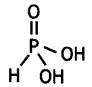Question 7.
Why does ammonia act as a Lewis base? (CBSE 2014)
Nitrogen atom in NH3 has one lone pair of electrons which is available for donation. Therefore,it acts as a Lewis base.

Question 8.
What is the oxidation number of phosphorus in H3PO2 molecule? (CBSE Delhi 2010)
+1

Question 9.
Out of white phosphorus and red phosphorus, which one is more reactive and why? (CBSE 2015)
White phosphorus is more reactive because of angular strain in the P4 molecules where the angles are only 60°.

Question 10.
What is the basicity of H3PO4? (CBSE Delhi 2015)
Basicity of H3PO4 is three because it has three ionisable hydrogen atoms.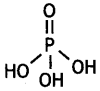Question 11.
On heating Pb(NO3)2 a brown gas is evolved which undergoes dimerisation on cooling. Identify the gas. (CBSE 2016)
NO2.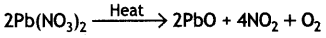2NO2 → N2O4

Question 12.
Solid PCl5 is ionic in nature. Give reason. (CBSE AI 2016)
PCl5 is ionic in the solid state because it exists as [PCl4]+ [PCl6] in which the cation is tetrahedral and anion is octahedral.

Question 13.
Write the structural difference between white P and red P. (CBSE Delhi 2014)
White P consists of P4 units in which four P atoms lie at the corners of a regular tetrahedron with ∠PPP = 60°. Red P also consists of P4 tetrahedra units but it has polymeric structure consisting of P4 tetrahedra linked together by covalent bonds.

Question 14.
What is the basicity of H3PO2 acid and why? (CBSE AI 2012)
H3PO2 has the structure:It has only one ionisable hydrogen and therefore, its basicity is one.

Question 15.
Name the promoter used In Haber’s process. (CBSE Sample Paper 2017-18)
Molybdenum.

Question 16.
NF3 is an exothermic compound whereas NCl3 is not. Explain. (CBSE AI 2011, 2012)
NF3 is an exothermic compound while NCl3 is not because:

• The bond dissociation enthalpy of F2 is lower than that of Cl2.
• Size of F is small as compared to Cl and therefore F forms stronger bonds with nitrogen releasing large amount of energy.

Therefore, overall energy is released during the formation of NF3 and energy is absorbed during the formation of NCl3.

Question 17.
N-N single bond is weaker than P-P single bond. (CBSE Delhi 2014)
N-N single bond is weaker than P-P single bond because of high interelectronic repulsions of non-bonding electrons due to small bond length.

Question 18.
What happens when orthophosphorous acid is heated? (CBSE Sample Paper 2017-18)
Phosphoric acid and phosphine are formed.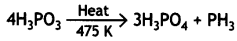Question 19.
Write the order of thermal stability of the hydrides of group 16 elements. (CBSE Delhi 2017)
The thermal stability of the hydrides of group 16 elements decreases from H2O to H2Te as:
H2O > H2S > H2Se > H2Te

Question 20.
The two O – O bond lengths in ozone molecule are equal. Why? (CBSE AI 2013, CBSE Delhi 2014)
Ozone is a resonance hybrid of two structures and therefore, the two O-O bond lengths are equal.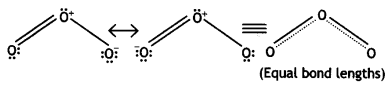Question 21.
SF6 is not easily hydrolysed. (CBSE Delhi 2005)
OR
SF6 is kinetically inert substance. Explain. (CBSE Al 2011, CBSE Delhi 2011)
SF6 is chemically inert and therefore, does not get hydrolysed. Its inert nature is due to the presence of stearically protected sulphur atom which does not allow thermodynamically favourable hydrolysis reaction.

Question 22.
Which of the following compounds has a lone pair of electrons at the central atom? (CBSE Sample Paper 2011)
H2S2O8, H2S2O7, H2SO3, H2SO4.
H2SO3

Question 23.
Ozone is thermodynamically unstable. Explain. (CBSE Sample Paper 2011)
Ozone is thermodynamically unstable with respect to oxygen because it results in liberation of heat (∆H is -ve) and increase in entropy (∆S is +ve). These two factors reinforce each other resulting negative ∆G (∆G = ∆H – T∆S) for its conversion to oxygen.Question 24.
Which neutral molecule would be isoelectronic with ClO? Is that molecule a Lewis base? (CBSE Delhi 2008)
ClO is isoelectronic with ClF. Yes, it is a Lewis base.

Question 25.
Which compound of xenon has distorted octahedral shape? (CBSE Sample Paper 2012)
XeF6 has distorted octahedral shape.

Question 26.
Iodide ions can be oxidised by oxygen in acidic medium. Give chemical equation to support this. (CBSE Sample Paper 2011)
Iodide can be oxidised by oxygen in acidic medium:
4I(aq) + O2(g) + 4H+(oq) > 2l2(s) + 2H2O(l).

Question 27.
What happens when XeF6 undergoes complete hydrolysis? (CBSE Sample Paper 2017-18)
XeO3 is formed.
XeF6 + 3H2O → XeO3 + 6HF

Question 28.
Which xenon compound is isostructural with lCl4? (CBSE Sample Paper 2011)
XeF4

Question 29.
Why conductivity of silicon increases on doping with phosphorus? (CBSE Delhi 2019)
When silicon is doped with phosphorus, an extra electron is introduced after forming four covalent bonds. This extra electron gets delocalised and serves to conduct electricity. Hence, conductivity increases.

### The p-Block Elements Important Extra Questions Short Answer Type

Question 1.
What inspired N. Bartlett for carrying out reaction between Xe and PtF6? (CBSE Delhi 2013)
In 1962, N. Bartlett noticed that platinum hexafluoride PtF6, is a powerful oxidising agent which combines with molecular oxygen to form ionic compound, dioxygenyl hexafluoroplatinate (V), O2+ [PtF6].
O2(S) + PtF6(g) → O2+ [PtF6]
This indicates that PtF6 has oxidised O2 to O2+. Now, oxygen and xenon have some similarities:

• The first ionisation energy of xenon gas (1170 kJ mol-1) is fairly close to that of oxygen (1177 kJ mol-1).
• The molecular diameter of oxygen and atomic radius of xenon are similar (4Å). These prompted Bartlett to carry out the reaction between Xe and PtF6.

Question 2.
Calculate the number of lone pairs on central atom in the following molecule and predict the geometry. (CBSE Sample Paper 2019)
XeF4 has square planar structure. It involves sp3d2 hybridisation of Xenon in which two positions are occupied by lone pairs.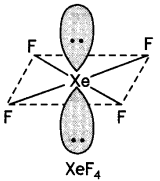Structure of XeF4 (Square planar)

Question 3.
How are interhalogen compounds formed? What general compositions can be assigned to them? (CBSE AI 2013)
The compounds containing two or more halogen atoms are called interhalogen compounds. They may be assigned general composition as XX’n, where X is halogen of larger size and X’ is of smaller size and X’ is more electronegative than X, e.g. ClF, ICl3, BrF5, IF7, etc.

Question 4.
Though nitrogen exhibits +5 oxidation state, it does not form pentahalides. Give reason. (CBSE 2013, CBSE Delhi 2015)
Nitrogen belongs to second period (n = 2) and has only s and p-orbitals. It does not have d-orbitals in its valence shell and therefore, it cannot extend its octet. That is why nitrogen does not form pentahalides.

Question 5.
Why does NO2 dimerise? Explain. (CBSE 2014, CBSE Delhi 2014)
NO2 contains odd number of valence electrons. It behaves as a typical molecule. In the liquid and solid state, it dimerises to form stable N2O4 molecule, with even number of electrons. Therefore, NO2 is paramagnetic, while N2O4 is diamagnetic in which two unpaired electrons get paired.

Question 6.
Draw the structure of O3 molecule. (CBSE Delhi 2010)
Ozone has angular structure as: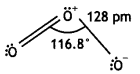It is resonance hybrid of the following structures:Question 7.
Complete the following equations: (CBSE 2014)
(i) Ag + PCl5
(ii) CaF2 + H2SO4
(i) 2Ag + PCl5 → 2AgCl + PCl3
(ii) CaF2 + H2SO4 → CaSO4 + 2HF

Question 8.
Write balanced chemical equations for the following processes:
(i) XeF2 undergoes hydrolysis.
(ii) MnO2 is heated with cone. HCl.
OR
Arrange the following in order of property indicated for each set:
(i) H2O, H2S, H2Se, H2Te – increasing acidic character
(ii) HF, HCl, HBr, HI – decreasing bond enthalpy (CBSE Delhi 2019)
(i) 2XeF2 + 2H2O → 2Xe + 4HF + O2
(ii) MnO2 + 4HCl → MnCl2 + 2H2O + Cl2
OR
(i) H2O < H2S < H2Se < H2Te
(ii) HF > HCI > HBr > HI

Question 9.
Explain the following giving an appropriate reason in each case.
(i) O2 and F2 both stabilise higher oxidation states of metals but O2 exceeds F2 in doing so.
(ii) Structures of xenon fluorides cannot be explained by Valence Bond approach. (CBSE 2012)
(i) This is because fluorine can show only -1 oxidation state, whereas oxygen can show -2 oxdiation state as well as positive oxidation states such as +2, +4 and +6.

(ii) Structures of xenon fluorides cannot be explained by Valence Bond approach because xenon is a noble gas and its octet is complete. Therefore, according to Valence Bond theory, xenon should not combine with fluorine to form fluorides due to its stable outermost shell configuration.

Question 10.
Draw the structures of the following molecules:
(i) XeOF4
(ii) H3PO3 (CBSE 2013)
(i)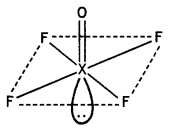Structure of XeOF4

(ii)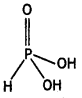Structure of H3PO3

Question 11.
How are interhalogen compounds formed? What general compositions can be assigned to them? (CBSE 2013)
The compounds containing two or more halogen atoms are called interhalogen compounds. They may be assigned general composition as XX’n, where X is halogen of larger size and X’ is of smaller size and X’ is more electronegative than X,
e.g. ClF, ICl3, BrF5, IF7, etc.

Question 12.
Draw the structures of the following molecules: (CBSE 2013)
(i) N2O5
(ii) XeF2
(i)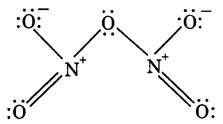(ii)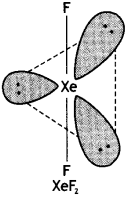Structure of XeF2 (Linear)

Question 13.
What happens when
(i) PCl5 is heated?
(ii) H3PO3 is heated?
Write the reactions involved. (CBSE Delhi 2013)
(i) On heating, PCl5 sublimes and decomposes on strong heating:(ii) On heating, H3PO3 decomposes into phosphoric acid and phosphine.
4H3PO3 → 3H3PO4 + PH3

Question 14.
Complete the following chemical equations:
(i) Ca3P2 + H2O →
(ii) Cu + H2SO4(conc.) →
OR
Arrange the following in the order of property indicated against each set:
(i) HF, HCl, HBr, HI – increasing bond dissociation enthalpy.
(ii) H2O, H2S, H2Se, H2Te – increasing acidic character. (CBSE Delhi 2014)
(i) Ca3P2 + 6H2O → 3Ca(OH)2 + 2PH3
(ii) Cu + 2H2SO24;(conc.) → CuSO4
+ SO2 + 2H2O
OR
(i) HI < HBr < HCl < HF
(ii) H2O < H2S < H2Se < H2Te

Question 15.
Complete the following equations:
(i) P4 + H2O →
(ii) XeF4 + O2F2 → (CBSE 2014)
(i) P4 + H2O → no reaction
(ii) XeF4 + O2F2 → XeF6 + O6

Question 16.
Draw the structures of the following:
(i) XeF2
(ii) BrF3
(i)Structure of XeF2 (Linear)

(ii)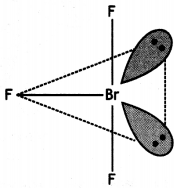Structure of BrF3 (T Shape)

Question 17.
(a) Draw the structure of XeF4.
(b) What happens when CaF2 reacts with cone. H2SO4? Write balanced chemical equation. (CBSE 2019C)
(a)(b) HF is formed.
CaF2 + H2SO4 → 2HF + CaSO4

Question 18.
(a) Draw the structure of H2S2O7.
(b) What happens when carbon reacts with cone. H2SO4? Write balanced chemical equation. (CBSE 2019C)
(a)(b) Carbon dioxide is formed.
C + 2H2SO4(conc.) → CO2 + 2 SO2 + 2H2O

Question 19.
Draw the structures of the following molecules: (CBSE 2014)
(i) XeO2
(ii) H2SO4
(i)(ii)Question 20.
Write the structure of the following: (HPO3)3 (CBSE 2016)
(HPO3)3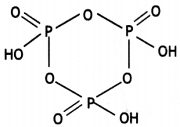Question 21.
Why is N2 less reactive at room temperature? (CBSE Al 2015, H.P.S.B. 2015, 2016, Meghalaya S.B. 2017)
In molecular nitrogen, there is a triple bond between two nitrogen atoms (NsN) and it is non-polar in character. Due to the presence of a triple bond, it has very high bond dissociation energy (941.4 kJ mol-1) and therefore, it does not react with other elements under normal conditions and is very unreactive. However, it may react at higher temperatures.

Question 22.
Phosphine has lower boiling point than ammonia. Give reason. (CBSE Al 2008,
CBSE Delhi 2013)
Ammonia exists as associated molecule due to its tendency to form hydrogen bonding. Therefore, it has high boiling point. Unlike NH3, phosphine (PH3) molecules are not associated through hydrogen bonding in liquid state.
This is because of low electronegativity of P than N. As a result, the boiling point of PH3 is lower than that of NH3.

Question 23.
Draw structures of the following:
(a) XeF4
(b) S2O82- (CBSE AI 2019)
(a)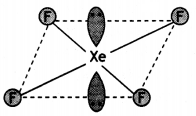(b)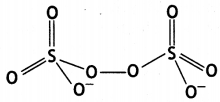Question 24.
(i) Draw the structure of phosphinic acid (H3PO2).
(ii) Write a chemical reaction for its use as reducing agent. (CBSE Sample Paper 2011)
(i) Phosphinic acid, H3PO2.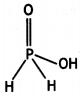(ii) H3PO2 reduces Ag+ ion to Ag which shows its reducing nature.
H3PO2 + 4AgNO3 + 2H2O → 4Ag + 4HNO3 + H3PO4

Question 25.
Among the hydrides of Group 15 elements, which have the
(a) lowest boiling point?
(b) maximum basic character?
(c) highest bond angle?
(d) maximum reducing character? (CBSE AI 2018)
(a) PH3
(b) NH3
(c) NH3
(d) BiH3

Question 26.
The bond angles (O-N-O) are not of the same value in NO2 and NO2+. Give reason. (CBSE Delhi 2012)
In NO2 there is one electron on N. Therefore, in NO2+, there is no electron on N atom and there is a lone pair of electrons on N atom in NO2.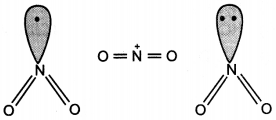Question 27.
H2PO2 is a stronger reducing agent than H3PO3. Give reason. (CBSEAI 2014, 2016)
The reducing character of the acid is due to H atoms bonded directly to P atom. In H3PO2, there are two P-H bonds whereas in H3PO3, there is one P-H bond. Therefore, H3PO2 is a stronger reducing agent than H2PO3.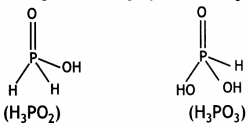Question 28.
Bi(V) is a stronger oxidising agent than Sb(V). Give reason. (CBSE Delhi 2014)
On moving down the group, the stability of +5 oxidation state decreases while the stability of +3 oxidation state increases due to inert pair effect. Therefore, +5 oxidation state of Bi is less stable than +5 oxidation state of Sb. Thus, Bi(V) is a stronger oxidising agent than Sb(V).

Question 29.
Dioxygen is a gas while sulphur is a solid at room temperature. Why?
(CBSE AI 2013, 2018)
Oxygen exists as a stable diatomic molecule and is, therefore, a gas. On the other hand, sulphur exists in solid state as S8 molecules and have puckered ring structure. The main reason for this different behaviour is that oxygen atom has strong tendency to form multiple bonds with itself and forms strong O=O bonds rather than O-O bonds.

On the other hand, sulphur-sulphur double bonds (S=S) are not very strong. As a result, catenated -O-O-O- chains are less stable as compared to O=O molecule while catenated -S-S-S- chains are more stable as compared to S = S molecule.
Therefore, oxygen exists as a diatomic gas and sulphur exists as S8 solid.

Question 30.
Draw structures of the following:
(a) H2S2O7
(b) HClO3 (CBSE Al 2019)
(a) H2S2O7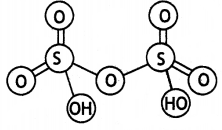(b) HClO3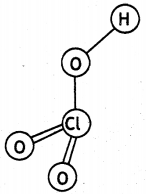Question 31.
Complete and balance the following equations:
(a) C + H2SO4 (cone.) →
(b) XeF2 + PF5
OR
Write balanced chemical equations involved in the following reactions:
(a) Fluorine gas reacts with water.
(b) Phosphine gas is absorbed in copper sulphate solution. (CBSE AI 2019)
(a)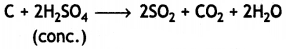(b)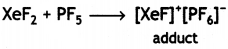OR

(a) 2F2 + 2H2O → 4HF + O2

(b)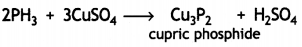Question 32.
Sulphuric acid has low volatility. Give chemical reactions in support of this. (CBSE Sample Paper 2011)
Sulphuric acid has low volatility and decomposes the salts of volatile acids forming its own salts:
2MX + H2SO4 → 2HX + M2SO4
(M = metal, X = F, Cl, NO3)
e.g.,NaCl + H2SO4 → NaHSO4 + HCl
KNO3 + H2SO4 → KHSO4 + HNO3.

Question 33.
Electron gain enthalpies of halogens are largely negative. Why? (CBSE AI 2017)
The halogens have the smallest size in their respective periods and therefore, high effective nuclear charge. Moreover, they have only one electron less than the stable noble gas configuration (ns2np6).

Therefore, they have strong tendency to accept one electron to acquire noble gas electronic configurations and hence have largely negative electron gain enthalpies.

Question 34.
Why does R3P = 0 exist but R3N = 0 does not (R = alkyl group). (CBSE AI 2014)
R3N = 0 does not exist because nitrogen cannot have covalency more than four. Moreover, R3P = 0 exists because phosphorus can extend its covalency more than 4 as well as it can form dπ-pπ bond whereas nitrogen cannot form dπ-pπ bond.

Question 35.
Write any two oxoacids of sulphur and draw their structures. (CBSE Al 2019)
(i) Sulphuric acid(ii) Peroxodisulphuric acid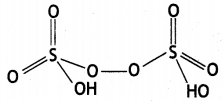Question 36.
Complete and balance the following equations:
(a) NH3 (excess) + Cl2
(b) XeF6 + 2H2O →
OR
Write balanced chemical equations involved in the following reactions:
(a) XeF4 reacts with SbF5.
(b) Ag is heated with PCl5. (CBSE AI 2019)
(a)
8NH3 + 3Cl2 → 6NH4Cl + N2
excess
(b) XeF6 + 2H2O → XeO2F2 + 4HF

OR

(a) XeF4 + SbF5 → [XeF3]+[SbF6]
(b) 2Ag + PCl5 → 2AgCl + PCl3

Question 37.
Complete and balance the following equations:
(a) S + H2SO4 (cone.) →
(b) PCl3 + H2O →
Write balanced chemical equations involved in the following reactions:
(a) Chlorine gas reacts with cold and dilute NaOH
(b) Calcium phosphide Is dissolved in water. (CBSE AI 2019)
(a) S + 2H2SO4(conc.) → 3SO2 + 2H2O
(b) PCl3 +3H2O → H3PO3 + 3HCl
OR
(a) Cl2 + ZNaOH → NaCl + NaOCl + H2O
(b) Ca3P2 + 6H2O → 3Ca(OH)2 + 2PH3

Question 38.
Bond enthalpy of fluorine Is lower than that of chlorine. Why? (CBSE AI 2018)
Fluorine atoms are smalL and internuclear distance between two F atoms In F2 molecule is also small (1 .43Å). As a result, electron-electron repulsions among the lone pairs on two fluorine atoms in F2 molecule are large. These Llarge electron-electron repulsions weaken the F-F bond. However, the electron-electron repulsions among lone pairs in Cl2 molecule are less because of Larger Cl atoms and larger Cl-Cl distance (1.99Å). Therefore, the bond enthalpy of fluorine is lower than that of chlorine.

### The p-Block Elements Important Extra Questions Long Answer Type

Question 1.
Give reasons for the following:
(a) Dioxygen is a gas but sulphur a solid.
(b) NO (g) released by jet aeroplanes is slowly depleting the ozone layer.
(c) Interhalogens are more reactive than pure halogens. (CBSE Al 2019)
(a) Due to small size and high electronegativity, oxygen atom forms pπ-pπ double bond, O = O. The intermolecular forces in oxygen are weak van der Waal’s forces and therefore, oxygen exists as a gas. On the other hand, sulphur does not form stable pπ-pπ bonds and does not exist as S2. It is linked by single bonds and forms polyatomic complex molecules having eight atoms per moledule (S8) and has puckered ring structure. Therefore, S atoms are strongly held together and it exists as a solid.

(b) Nitrogen oxide (NO) gas emitted from the exhaust of jet aeroplanes readily combines with ozone to form nitrogen dioxide and diatomic oxygen.
NO + O3 → NO2 + O2
Since jet aeroplanes fly in the stratosphere, near ozone layer, these are responsible for the depletion of ozone layer.

(c) This is because covalent bond between dissimilar atoms in interhalogens (X- Y) is polar and weaker than between similar atoms (X-X and Y-Y). This is due to the fact that the overlapping of orbitals of dissimilar atoms is less effective than the overlapping between similar atoms.

Question 2.
Give reasons for the following:
(i) Where R is an alkyl group, R3P = 0 exists but R3N = 0 does not.
(ii) PbCl4 is more covalent than PbCl2.
(iii) At room temperature, N2 is much less reactive. (CBSE AI 2013)
(i) R3N = 0 does not exist because nitrogen cannot have covalency more than four. But R3P = 0 exists because phosphorus can extend its covalency more than 4 as well as it can form dπ-pπ bonds whereas nitrogen cannot form dπ-pπ bond.

(ii) Pb4+ has smaller size than Pb2+. As a result, it can polarise the Cl ion to greater extent than Pb2+. Consequently, PbCl4 is more covalent than PbCl2.

(iii) In molecular nitrogen, there is a triple bond between two nitrogen atoms (N = N) and it is non-polar in character. Due to the presence of a triple bond, it has very high bond dissociation energy (941.4 kJ mol-1) and therefore, it does not react with other elements under normal conditions and is very unreactive. However, it may react at higher temperatures.

Question 3.
Give reasons for the following:
(i) Oxygen is gas but sulphur is a solid,
(ii) O3 acts as a powerful oxidising agent.
(iii) BiH3 is the strongest reducing agent amongst all the hydrides of Group 15 elements. (CBSE Al 2013)
(i) Oxygen exists as a stable diatomic molecule and is, therefore, a gas.
On the other hand, sulphur exists in solid state as S8 molecules and have puckered ring structure. The main reason for this different behaviour is that oxygen atom has good tendency to form multiple bonds with itself and forms strong o = o bonds than o – o bonds. On the other hand, sulphur- sulphur double bonds (S = S) are not very strong. As a result, catenated -O-O-O- chains are less stable as compared to O = O molecule while catenated -S-S-S- chains are more stable as compared to S = S molecule. Therefore, oxygen exists as a diatomic gas and sulphur exists as S8 solid.

(ii) Ozone acts as a powerful oxidising agent because it has higher energy content and decomposes readily to give atomic oxygen as:
O3 → O2 + O
Therefore, ozone can oxidise a number of non-metals and other compounds. For example,
PbS (s) + 4O3 (8) → PbSO4 (s) + 4O2 (g)
2I (aq) + H2O(l) + O3(g) → 2OH (aq) + I32(s) + O2(g)

(iii) The stability of group 15 hydrides decreases from NH3 to BiH3. Therefore, BiH3 is most unstable hydride among group 15 hydrides because of lowest M-H bond strength. As a result, BiH3 is the strongest reducing agent.

Question 4.
Give reasons for the following:
(i) Though nitrogen exhibits +5 oxidation state, it does not form pentahalide.
(ii) Electron gain enthalphy with negative sign of fluorine is less than that of chlorine.
(iii) The two oxygen-oxygen bonds lengths in ozone molecule are identical. (CBSE Al 2013)
(i) Nitrogen does not have vacant d-orbitals in its valence shell. Therefore, it cannot extend its valency beyond 3. Thus, it cannot form pentahalides.

(ii) The less negative electron gain enthalpy of fluorine as compared to chlorine is due to very small size (72 pm) of fluorine atom. As result, there are strong inter- electronic repulsions in the relatively small 2p sub-shell of fluorine and therefore, the incoming electron does not feel much attraction. Thus, its electron gain enthalpy is small.

(iii) Ozone is resonance hybrid of two structures and therefore, the two oxygen-oxygen bonds are identical.Question 5.
How would you account for the following:
(i) H2S is more acidic than H2O.
(ii) The N-O bond in NO2 is shorter than the N-O in NO3.
(iii) Both O2 and F2 stabilise higher oxidation states but the ability of oxygen to stabilise the higher oxidation states exceeds that of fluorine. (CBSE 2011)
(i) Since size of S is more than that of O, the distance between central atom and hydrogen atoms is more in H2S than in H2O. Therefore, bond dissociation enthalpy of H2S is less and bond cleavage is more easy than in H2O. Therefore, H2S is more acidic than H2O.

(ii) In the resonance structures of NO2, two bonds are sharing a double bond, while in NO3, three bonds are sharing a double bond.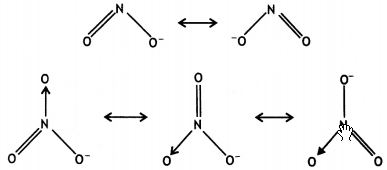As a result, the bond in NO2 will be shorter than in NO3.

(iii) The larger tendency of oxygen to stabilise the higher oxidation state is because of the tendency of oxygen to form multiple bonds with metal.

Question 6.
How would you account for the following:
(i) NF3 is an exothermic compound but NCl3 is not.
(ii) The acidic strength of compounds increases in the order:
PH3 < H2S < HCl
(iii) SF6 is kinetically inert. (CBSE 2011)
(i) NF3 is an exothermic compound while NCl3 is an endothermic compound because
(a) The bond dissociation enthalpy of F2 is lower as compared to Cl2.
(b) Size of F is small as compared to Cl and therefore, F forms stronger bond with N releasing large amount of energy.
Therefore, overall energy is released during the formation of NF3 and energy is absorbed during the formation of NCl3.

(ii) The bond dissociation enthalpy decreases in the order:
P-H > S-H > Cl-H
Therefore, acidic character follows the order:
PH3 < H2S < HCl

(iii) SF6 is kinetically inert because sulphur is sterically protected by six F atoms and it is a coordinatively saturated compound. Therefore, it does not undergo thermodynamically favourable hydrolysis reaction.

Question 7.
(a) Draw the structures of the following molecules:
(i) XeOF4
(ii) H2SO4
(b) Write the structural difference between white phosphorus and red phosphorus. (CBSE Delhi 2014)
(a) (i) XeOF4(ii) H2SO4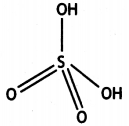(b) White phosphorus consists of P4 units in which four P atoms lie at the corners of a regular tetrahedron with ΔPPP = 60°.
Red phosphorus also consists of P4 tetrahedra units, but it has polymeric structure consisting of P4 tetrahedra linked together by covalent bond.

Question 8.
Account for the following:
(i) Bi(V) is a stronger oxidising agent than Sb(V).
(ii) N-N single bond is weaker than P-P single bond.
(iii) Noble gases have very low boiling points. (CBSE Delhi 2014)
(i) On moving down the group, the stability of +5 oxidation state decreases while the stability of +3 oxidation state increases due to inert pair effect. Therefore, +5 oxidation state of Bi is less stable than +5 oxidation state of Sb. Thus, Bi(V) is a stronger oxidising agent than Sb(V).

(ii) N-N single bond is weaker than single P-P bond because of high interelectronic repulsions of non-bonding electrons due to small bond length.

(iii) Noble gases are monoatomic gases and are held together by weak vander Waals forces. Therefore, these are liquified at very low temperatures. Hence they have low boiling points.

Question 9.
Give reasons for the following: (CBSE 2014)
(i) (CH3)3 P = 0 exists but (CH3)3 N = 0 does not.
(ii) Oxygen has less electron gain enthalpy with negative sign than sulphur.
(iii) H3PO2 is a stronger reducing agent than H3PO3.
(i) (CH3)3 N = 0 does not exist because
nitrogen cannot have covalency more than four. Moreover, (CH3)3 P = 0 exists because phosphorus can extend its covalency more than 4 as well as it can form dπ – pπ bonds whereas nitrogen cannot form dπ – pπ bonds.

(ii) This is due to small size of oxygen atom so that its electron cloud is distributed over a small region of space and therefore, it repels the incoming electron. Hence the electron gain enthalpy of oxygen is less negative than sulphur.

(iii) In H3PO3, there are two P-H bonds whereas in H3PO3, there is one P-H bond. Therefore, H3PO3 is stronger reducing agent than H3PO3.Question 10.
Assign reason for the following:
(i) H3PO2 is a stronger reducing agent than H3PO4.
(ii) Sulphur shows more tendency for catenation than oxygen.
(iii) Reducing character increases from HF to HI. (CBSE 2016)
(i) The reducing character of the acid is due to H atoms bonded directly to P atom. In H3PO2, there are two P-H bonds whereas in H3PO3, there is one P-H bond. Therefore, H3PO2 is a stronger reducing agent than H3PO3.(ii) Sulphur shows more tendency for catenation than oxygen because of stronger S-S bonds than O-O bonds.

(iii) Due to decrease in bond enthalpy down the group, the thermal stability decreases from HF to HI.
As a result of decrease in stability from HF to HI, the reducing character increases down the group as:
HF < HCl < HBr < HI

Question 11.
What happens when
(i) (NH4)2Cr2O7 is heated?
(ii) PCl5 is heated?
(iii) H3PO3 is heated? Write the equations involved. (CBSE AI 2015, CBSE Delhi 2008, 2013, 2017)
(i) On heating (NH4)2Cr2O7, nitrogen gas is evolved.(ii) On heating, PCl5 first sublimes and then decomposes on strong heating: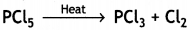(iii) On heating, H3PO3 disproportionates to give orthophosphoric acid and phosphine.Question 12.
(a) Suggest a quantitative method for estimation of the gas which protects us from U.V. rays of the sun.
(b) Nitrogen oxides emitted from the exhaust system of supersonic jet aeroplanes slowly deplete the concentration of ozone layer in upper atmosphere. Comment. (CBSE Sample Paper 2011)
(a) The gas which protects us from U.V. rays of the sun is ozone. It reacts with I ions to give iodine as:
O3 + 2I + H2O → O2 + l2 + 2OH
l2 liberated is titrated against sodium thiosulphate solution and amount of O3 can be estimated.

(b) The release of nitrogen oxides (NOx) into stratosphere by the exhaust system of supersonic jet aeroplanes deplete the concentration of O2 because NO reacts with O3 to give O2.
NO (g) + O2 (g) → NO2 (S) + O2 (s)
Therefore, NO is slowly depleting the concentration of ozone.

Question 13.
Give reasons for the following:
(a) Acidic character decreases from N2O3 to Bi2O3.
(b) All the P-Cl bonds in PCl5 are not equivalent.
(c) HF is a weaker acid than HCl in an aqueous solution. (CBSE Al 2019)
(a) On moving down the group, metallic character increases. Therefore acidic character of oxides decreases from nitrogen to bismuth.

(b) PCl5 has trigonalbipyramidal geometry.In this geometry, axial bonds are larger than equatorial bonds because of large repulsions at axial positions. Therefore, all P-Cl bonds in PCl5 are not equivalent.

(c) The H-F bond has high bond dissociation enthalpy than H-Cl bond. Therefore, H-F bond is stronger and can be dissociated into H+ and F ions with difficulty. Hence, it is weaker acid than HCl.

Question 14.
Give reasons for the following:
(a) O-O single bond is weaker than S-S single bond.
(b) Tendency to show -3 oxidation state decreases from Nitrogen (N) to Bismuth (Bi).
(c) Cl2 acts as a bleaching agent. (CBSE AI 2019)
(a) O-O single bond is weaker than S-S single bond because oxygen has smaller size and has high inter-electronic repulsion.

(b) Tendency to show -3 oxidation state decreases because metallic character and size increase down the group.

(c) Cl2 acts as a bleaching agent in the presence of moisture
Cl2 + H2O → 2HCl + [O]
Coloured matter + [O] → Colourless matter
The nascent oxygen is responsible for the bleaching action of Cl2.

Question 15.
(a) Draw the structures of the following molecules:
(i) XeOF4
(ii) H2SO4
(b) Write the structural difference between white phosphorus and red phosphorus. (CBSE Delhi 2014)
(a) (i) XeOF4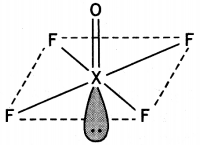(ii) H2SO4(b) White phosphorus consists of P4 units in which four P atoms lie at the corners of a regular tetrahedron with ΔPPP = 60°.
Red phosphorus also consists of P4 tetrahedra units, but it has polymeric structure consisting of P4 tetrahedra linked together by covalent bond.

Question 16.
Account for the following:
(i) PCl5 is more covalent than PCl3.
(ii) Iron on reaction with HCl forms FeCl2 and not FeCl3.
(iii) The two O-O bond lengths in the ozone molecule are equal. (CBSE Delhi 2014)
(i) In PCl5, the oxidation state of P is +5 while in PCl3, it is +3. As a result of higher oxidation state of the central atom, PCl5 has larger polarising power and can polarise the chloride ion (Cl) to a greater extent than in the corresponding PCl3. Since larger the polarisation, larger is the covalent character; PCl5 is more covalent than PCl3.

(ii) Iron reacts with HCl to form ferrous chloride with the evolution of hydrogen gas.
Fe + 2HCl → FeCl2 + H2

(iii) The two O-O bond lengths in ozone molecule are equal and are intermediate between single and double bonds. This is because of resonance between two structures (a) and (b) and actual molecule is resonance hybrid of these two structures: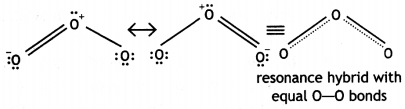Question 17.
Account for the following:
(a) Moist SO2 decolourises KMnO4 solution.
(b) In general, interhalogen compounds are more reactive than halogens (except fluorine).
(c) Ozone acts as a powerful oxidising agent. (CBSE Sample Paper 2019)
Moist sulphur dioxide behaves as a reducing agent. It reduces MnO4 to Mn2+.
5 SO2 + 2MnO4 + 2H2O → 5 SO42- + 4H+ + 2Mn2+

(b) X-X’ bond in inter halogens is weaker than X-X bond in halogens except F-F bond. This is due to the fact that the overlapping of orbitals of two dissimilar atoms is less effective than the overlapping of orbitals of similar atoms.

(c) Due to the ease with which it liberates atoms of nascent oxygen, Ozone acts as a powerful oxidising agent.
O3 → O2 + O

Question 18.
(a) What happens when
(i) chlorine gas is passed through a hot concentrated solution of NaOH?
(ii) sulphur dioxide gas is passed through an aqueous solution of Fe(lll) salt?
(a) (i) Sodium chlorate and sodium chloride are formed.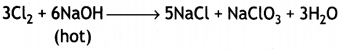(ii) Fe (III) salt is reduced to Fe (II) salt.
2Fe3+ + SO2 + 2H2O → 2Fe2+ + SO42- + 4H+

(i) What is the basicity of H3PO3 and why?
(ii) Why does fluorine not play the role of a central atom in interhalogen compounds?
(iii) Why do noble gases have very low boiling points? (CBSE Delhi 2011)
(i) H3PO3 is dibasic and has basicity of
two because it has two P-OH bonds which are ionisable. The third H atom is linked to P and is non-ionisable.H3PO3 ⇌ HPO32- + 2H+

(ii) Fluorine does not play the role of a central atom in interhalogen compounds because it is highly electronegative. Moreover, it has only one electron less than the octet and does not have vacant d-orbitals in its valence shell. Therefore, it can form only one bond with other halogen atoms and cannot act as central atom in interhalogen compounds.

(iii) Noble gases have very low boiling points because only weak van der Waals’ forces are present between the atoms of the noble gases in the liquid state.

Question 19.
(a) Give reasons for the following:
(i) Bond enthalpy of F2 is lower than that of Cl2.
(ii) PH3 has lower boiling point than NH3.
(a) (i) Due to small size of F atom, there are strong repulsions between the non-bonding electrons of F atoms in the small sized F2 molecule. Therefore, bond enthalpy of F2 is lower than relatively larger Cl2 molecule in which repulsions between non-bonding electrons are less.

(ii) Ammonia exists as associated molecules due to its tendency to form hydrogen bonding. Therefore, it has high boiling point. Unlike NH3, phosphine (PH3) molecules are not associated through hydrogen bonding in liquid state. This is because of low electronegativity of P than N. As a result, the boiling point of PH3 is lower than that of NH3.

(b) Draw the structures of the following molecules:
(i) BrF3
(ii) (HPO3)3
(iii) XeF4
(i)BrF3 (T shape)

(ii)(HPO3)3

(iii)XeF4

OR

(a) Account for the following:
(i) Helium is used in diving apparatus.
(ii) Fluorine does not exhibit positive oxidation state.
(iii) Oxygen shows catenation behaviour less than sulphur.
(a) (i) Helium alongwith oxygen (helium- oxygen mixture) is used by deep sea divers in preference to nitrogen-oxygen mixture because of its low solubility in blood.

(ii) Fluorine is the most electronegative element and therefore, it shows an oxidation state of -1 only. It does not show any positive oxidation state.

(iii) Sulphur has strong tendency for catenation, i.e. forming bonds with itself in comparison to oxygen. This is because of stronger S-S single bond than O-O single bond.

(b) Draw the structures of the following molecules.
(i) XeF2
(ii) H2S2O8 (CBSE Delhi 2013)
(i)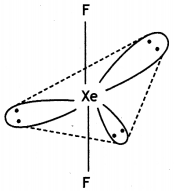XeF2 (Linear)

(ii)Question 20.
(a) Give reasons for the following:
(i) Sulphur in vapour state shows paramagnetic behaviour.
(ii) N-N bond is weaker than P-P bond.
(iii) Ozone is thermodynamically less stable than oxygen.
(a) (i) In the vapour state, sulphur exists as S2 molecules. S2 molecule has two unpaired electrons in anti-bonding molecular orbitals (πx and πy) and hence shows paramagnetism.

(ii) The N-N bond is weaker than P-P bond because of high interelectronic repulsions of non-bonding electrons due to small N-N bond length (109 pm).

(iii) Ozone is thermodynamically unstable with respect to oxygen because it results in liberation of heat (ΔH is negative) and increase in entropy (ΔS positive). These two factors make large negative ΔG for its conversion to oxygen.

(b) Write the name of gas released when Cu is added to
(i) dilute HNO3 and
(ii) conc. HNO3
(i) With dilute HNO3; NO (nitric oxide)
3Cu + 8HNO3(dil) → 3Cu(NO3)2 + 2NO + 4H2O

(ii) With cone. HNO3: NO2 (nitrogen dioxide)
Cu + 4HNO3(conc) → Cu(NO3)2 + 2NO2 + 2H2O

OR

(a) (i) Write the disproportionation reaction of H3PO3.
(ii) Draw the structure of XeF4.
(i) 4H3PO3 → 3H3PO4 + PH3

(ii)(b) Account for the following:
(i) Although fluorine has less negative electron gain enthalpy, yet F2 is strong oxidising agent.
(ii) Acidic character decreases from N2O3 to Bi2O3 in group 15.
(i) Fluorine is the strongest oxidising agent, although it has less negative electron gain enthalpy. This is because of its smaller size, large bond dissociation enthalpy of F2 and high exothermic hydration enthalpy of small F ion.

(ii) On moving down the group, the metallic character increases. Therefore, the acidic character of oxides decreases down the group from N2O3 to Bi2O3

(c) Write a chemical reaction to test sulphur dioxide gas. Write chemical equation involved. (CBSE Delhi 2019)
SO2 decolourises pink violet colour of acidified potassium permanganate solution.
2KMnO4 + 5SO2 + 2H2O → K2SO4 + 2MnSO4 + 2H2SO4

Question 21.
(a) Complete the following chemical reaction equations:
(i) P4 + SO2Cl4
(ii) XeF6 + H4O →
(i) P4 + 10SO2Cl2 → 4PCl5 + 10SO2

(ii) XeF6 + H2O → XeOF4 + 2HF

(b) Predict the shape and the asked angle (90° or more or less) in each of the following cases:
(i) SO32- and the angle O – S – O
(ii) ClF3 and the angle F – Cl – F
(iii) XeF2 and the angle F – Xe – F
(i) SO32-The O-S-O angle is more than 90°.

(ii) ClF3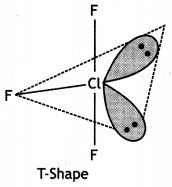The F-Cl-F bond angle is equal to 90°.

(iii)
XeF2F-Xe-F bond angle is equal to 180° (greater than 90°).

OR

(a) Complete the following chemical equations:
(i) NaOH + Cl2
(hot and cone.)
(ii) XeF4 + O2F2
(i) 6NaOH + 3Cl2 → 5NaCl + NaClO3 + 3H2O
(Hot and conc.)

(ii) XeF4 + O2F2 → XeF6 + O2

(b) Draw the structures of the following molecules:
(i) H3PO2
(ii) H2S2O7 (CBSE 2012)
(i) H3PO2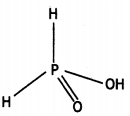(ii) H2S2O7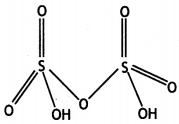Question 22.
(a) Draw the molecular structures of following compounds:
(i) XeF6
(ii) H2S2O8
(i)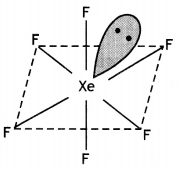(ii)(b) Explain the following observations:
(i) The molecules NH3 and NF3 have dipole moments which are of opposite directions.
(ii) All the bonds in PCl5 molecule are not equivalent.
(iii) Sulphur in vapour state exhibits paramagnetism.
(i) Both NH3 and NF3 have pyramidal shape with one lone pair on N atom.The lone pair on N is in opposite direction to the N-F bond moments and therefore, it has very low dipole moment (about 0.234 D). But ammonia has high dipole moment because its lone pair is in the same direction as the N-H bond moments.

(ii) PCl5 has trigonal bipyramidal structure in which there are three P-Cl equatorial bonds and two P-Cl axial bonds. The two axial bonds are being repelled by three bond pairs at 90° while the three equatorial bonds are being repelled by two bond pairs at 90°. Therefore, axial bonds are repelled more by bond pairs than equatorial bonds and hence are larger (219 pm).

(iii) In vapour state, sulphur partly exists as S2 molecule and S2 molecule like O2 has two unpaired electrons in anti-bonding π* molecular orbitals. Therefore, it is paramagnetic.

OR

(a) Complete the following chemical equations:
(i) XeF4 + SbF5
(ii) Cl2 + F2 (excess ) →
(i) XeF4 + SbF5 → XeF4.SbF5 → [XeF3]+ [SbF6]

(ii)(b) Explain each of the following:
(i) Nitrogen is much less reactive than phosphorus.
(ii) The stability of +5 oxidation state decreases down group 15.
(iii) The bond angles (O – N – O) are not of the same value in NO2 and NO2+. (CBSE Delhi 2012)
(i) In molecular nitrogen, there is a triple bond between two nitrogen atoms (N = N) and it is non-polar in character.
Due to the presence of a triple bond, it has very high bond dissociation energy (941.4 kJ mol-1) and therefore, it does not react with other elements under normal conditions and is very unreactive. However, it may react at higher temperatures.

(ii) The stablity of +5 oxidation state decreases down the group because of inert pair effect. Therefore, the +5 oxidation state of Bi is less stable than that of Sb.

(iii) In NO2, there is one electron on N while in NO2+ there is no electron and in NO2+, there is a lone pair of electrons on N as shown below: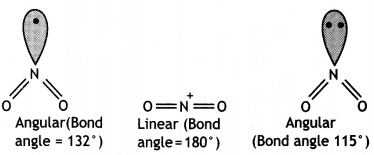NO2+ molecule is linear and has bond angle of 180°. The repulsion by single electron is less as compared to repulsion by a lone pair of electrons. Therefore, bond pairs in NO2 are forces more closer than in NO2.

Question 23.
(a) Draw the structure of the following molecule: H3PO2
(a)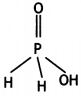(b) Explain the following observations:
(i) Nitrogen is much less reactive than phosphorus.
(ii) Despite having greater polarity, hydrogen fluoride boils at a lower temperature than water.
(iii) Sulphur has greater tendency for catenation than oxygen in the same group.
(i) In molecular nitrogen, there is a triple bond between two nitrogen atoms (N = N) and it is nonpolar in character.
Due to the presence of a triple bond, it has very high bond dissociation energy (941.4 kJ mol-1) and therefore, it does not react with other elements under normal conditions and is very unreactive. However, it may react at higher temperatures.

(ii) Though electronegativity of F is more than O, yet water forms more extensive hydrogen bonding than HF. In H2O, each oxygen is tetrahedrally surrounded by two covalent bonds and two hydrogen bonds, (-O….H). In HF there is only one hydrogen bond (-F….H).

(iii) Sulphur has strong tendency for catenation, i.e. forming bonds with itself in comparison to oxygen. This is because of stronger S-S single bond than O-O single bond.

OR

(a) Draw the structures of the following molecules:
(i) N2O5
(ii) HClO4

(a)
(i)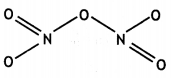(ii)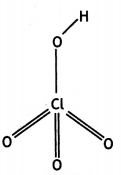(b) Explain the following observations:
(i) H2S is more acidic than H2O.
(ii) Fluorine does not exhibit any positive oxidation state.
(iii) Helium forms no real chemical compound. (CBSE 2012)
(i) The size of S is more than that of O. Therefore, the distance between S and H, i.e. S-H bond length, is more than O-H bond length. As a result, the bond dissociation enthalpy of S-H will be less and it will be easier to break the bond in H2S than O-H bond in water. Therefore, H2S will be more acidic than H2O.

(ii) Fluorine is the most electronegative element and therefore, it shows oxidation state of – 1 only. It does not show any positive oxidation state.

(iii) Helium does not form compounds because it has very high ionisation enthalpy and smallest size. Its electron gain enthalpy is also almost zero.

Question 24.
Write balanced equations for the following reactions:
(a) P4 + NaOH + H2O → CBSE Delhi 2009)
(b) As4 + Cl2(excess) →
(c) P4O10 + H2O →
(d) Ca3P2 + H2O → (CBSE Delhi 2008, 2014)
(e) POCl3 + H2O →
(f) HgCl2 + PH3 → (CBSE AI 2010)
(g) Ag + PCl5 → (CBSE AI 2014)
(a) P4 + 3NaOH + 3H2O → PH3 + 3NaH2PO2
(b) As4 + 10Cl4(excess) → 4AsCl5
(c) P4O10 + 6H4O → 4H3PO4
(d) Ca3P2 + 6H2O → 2PH3 + 3 Ca(OH)2
(e) POCl3 + 3H2O → H3PO4 + 3HCl
(f) 3HgCl2 + 2PH3 → Hg3P2 + 6HCl
(g) 2Ag + PCl5 → 2 AgCl + PCl3

Question 25.
Complete the following reactions
(i) NaOH + Cl2
hot and conc.
(ii) NH3 + Cl2 (excess) → (CBSE Delhi 2017)
(iii) NaNO2 + HCl →
(iv) F2(g) + H2O(l) → (CBSE Delhi 2008)
(v) K2CO3 + HCl →
(vi) Cl2 + H2O → (CBSE Delhi 2017)
(vii) F2 + 2Cl → (CBSE Delhi 2017)
(i) 6NaOH + 3Cl2 → 5NaCl + NaClO3 + 3H2O
hot and conc.
(ii) NH3 + 3Cl2 (excess) → NCl3 + 3HCl
(iii) 2NaNO2 + 2HCl → 2NaCl + H2O + NO2 + NO
(iv) 2F2(g) + 2H2O(l) → 4H+(aq) + 4F (aq) + O2(g)
(v) K2CO3 + 2HCl → 2KCl + CO2 + H2O
(vi) 2Cl2 + 2H2O → 4HCl + O2
(vii) F2 + 2Cl → 2F + Cl2

Question 26.
Complete the following reactions:
(i) XeF4 + SbF5 → (CBSE Delhi 2012)
(ii)(CBSE Delhi 2012, CBSE Al 2012, 2014)
(iii) XeF2 + H2O →
(iv) XeF4 + H2O →
(v) XeF6 + H2O → (CBSE Delhi 2012)
(vi) XeF6 + 2H2O → (CfiSE Delhi 2017)
(vii) XeF6 + 3H2O → (CBSE Delhi 2017)
(i) XeF4 + SbF5 → [XeF3]+ [SbF6]
(ii)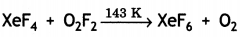(iii) XeF2 + H2O → 2Xe + 4HF + O2
(iv) XeF4 + H2O → 4Xe + 2XeO3 + 24HF + 3O2
(v) XeF6 + H2O → XeOF4 + 2HF
(vi) XeF6 + 2H2O → XeO2F2 + 4HF
(vii) XeF6 + 3H2O → XeO3 + 6HF

Question 27.
(a) Account for the following:
(i) Ozone is thermodynamically unstable.
(ii) Solid PCl5 is ionic in nature.
(iii) Fluorine forms only one oxoacid HOF.
(a) (i) Ozone is thermodynamically unstable with respect to oxygen because it results in liberation of heat (ΔH is -ve) and increase in entropy ΔS is +ve). These two factors reinforce each other resulting in negative ΔG (ΔG = ΔH – TΔS) for its conversion to oxygen.

(ii) PCl5 has trigonal bipyramidal structure and is not very stable. It splits up into more stable tetrahedral and octahedral structures which are stable as
PCl5 ⇌ [PCl4]+ [PCl6]
Therefore, it exists as ionic.

(iii) Due to small size and high electronegativity, fluorine cannot act as central atom in higher oxoacids.

(b) Draw the structure of
(i) BrF5
(ii) XeF4
(i)Square Pyramidal

(ii)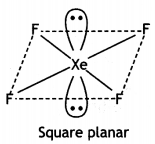OR

(i) Compare the oxidising action of F2 and Cl2 by considering parameters such as bond dissociation enthalpy, electron gain enthalpy and hydration enthalpy.
F2 is stronger oxidising agent than Cl2. This can be explained on the basis of bond dissociation enthalpy, electron gain enthalpy and hydration enthalpy.
The process of oxidising behaviour may be expressed as: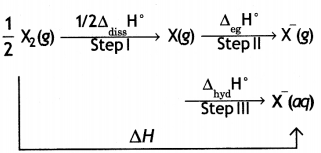The overall tendency for the change (i.e. oxidising behaviour) depends upon the net effect of three steps. As energy is required to dissociate or convert molecular halogen into atomic halogen, the enthalpy change for this step is positive. On the other hand, energy is released in steps (II) and (III), therefore, enthalpy change for these steps is negative.

Now although fluorine has less negative electron gain enthalpy, yet it is stronger oxidising agent because of low enthalpy of dissociation and very high enthalpy of hydration. In other words, large amount of energy released in step (III) and lesser amount of energy required in step (I) overweigh the smaller energy released in step (II) for fluorine. As a result, the AH overall is more negative for F2 than for Cl2. Hence, F2 is stronger oxidising agent than Cl2.

(ii) Write the conditions to maximise the yield of H2SO4 by contact process.
Conditions for maximum yield of H2SO4 by Contact Process:
(a) Low temperature (optimum temperature 720 K)
(b) High pressure (optimum pressure 2 bar)
(c) Presence of catalyst (V2O5 catalyst).

(iii) Arrange the following in the increasing order of property mentioned:
(a) H3PO3, H3PO4, H3PO2 (Reducing character)
(b) NH3, PH3, ASH3, SbH3, BiH3 (Base strength) (CBSE 2016)
(a) H3PO2 > H3PO3 > H3PO4
(b) NH3 > PH3 > ASH3 > SbH3 > BiH3

Question 28.
(a) Account for the following:
(i) Acidic character increases from HF to HI.
(ii) There is large difference between the melting and boiling points of oxygen and sulphur.
(iii) Nitrogen does not form pentahalide.
(a) (i) In gaseous state, hydrogen halides are covalent. But in aqueous solution, they ionise and behave as acids. The acidic strength of these acids decreases in the order:
HI > HBr > HCl > HF
Thus, HF is the weakest acid and HI is the strongest acid among these hydrogen halides.

The above order of acidic strength is reverse of that expected on the basis of electronegativity. Fluorine is the most electronegative halogen, therefore, the electronegativity difference will be maximum in HF and should decrease gradually as we move towards iodine through chlorine and bromine.

Thus, HF should be most ionic in nature and consequently it should be strongest acid. Although many factors contribute towards the relative acidic strengths, the major factor is the bond dissociation energy. The bond dissociation energy decreases from HF to HI so that HF has maximum bond dissociation energy and HI has the lowest value.

Since H-I bond is weakest, it can be dissociated into H+ and I ions readily while HF can be dissociated with maximum difficulty. Thus, HI is the strongest acid while HF is the weakest acid among the hydrogen halides.

(ii) Oxygen molecule is held by weak van der Waals forces because of the small size and high electronegativity of oxygen. On the other hand, sulphur molecules do not exist as S2 but form polyatomic molecules having eight atoms per molecule (S8) linked by single bonds. Therefore, S atoms are strongly held together by intermolecular forces and its melting point is higher than that of oxygen. Hence, there is large difference in melting and boiling points of oxygen and sulphur.

(iii) In molecular nitrogen, there is a triple bond between two nitrogen atoms (N ≡ N) and it is non-polar in character. Due to the presence of a triple bond, it has very high bond dissociation enthalpy (941.4 kJ mol-1) and therefore, it does not react with other elements under normal conditions and is very unreactive. However, it may react at higher temperatures.

(b) Draw the structures of the following:
(i) CIF3
(ii) XeF4
(i)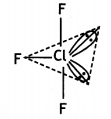T – Shaped molecule

(ii)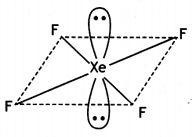Square planar molecule

OR

(i) Which allotrope of phosphorus is more reactive and why?
White phosphorus is most reactive of all the allotropes because it is unstable due to angular strain on P4 molecule with bond angle of 60°.

(ii) How are the supersonic jet aeroplanes responsible for the depletion of ozone layers?
Nitrogen oxide emitted from exhausts of supersonic jet aeroplanes readily combines with ozone to form nitrogen dioxide and diatomic oxygen. Since supersonic jets fly in the stratosphere near ozone layer, they are responsible for the depletion of ozone layer.

(iii) F2 has lower bond dissociation enthalpy than Cl2. Why?
Because of small size of fluorineatoms, there are strong electron-electron repulsions between the lone pairs of electrons on F atoms. Hence, bond dissociation enthalpy of F2 is lower than that of Cl2.

(iv) Which noble gas is used in filling balloons for meteorological observations?
Helium is used for filling balloons for meteorological observations because it is non-inflammable.

(v) Complete the equation: (CBSE 2015)
XeF2 + PF5
XeF2 + PF5 → [XeF]+ [PF6]

Question 29.
(a) Account for the following:
(i) Interhalogens are more reactive than pure halogens.
(ii) N2 is less reactive at room temperature.
(iii) Reducing character increases from NH3 to BiH3.
(i) Interhalogen compounds are more reactive than component halogens.
This is because covalent bond between dissimilar atoms in interhalogen compounds is polar and weaker than between similar atoms in halogens (except F-F). This is due to the fact that the overlapping of orbitals of dissimilar atoms is less effective than the overlapping of orbitals of similar atoms.

(ii) In molecular nitrogen, there is a triple bond between two nitrogen atoms (N ≡ N) and it is non-polar in character. Due to the presence of a triple bond, it has very high bond dissociation energy (941.4 kJ mol-1) and therefore, it does not react with other elements under normal conditions and is very unreactive. However, it may react at higher temperatures.

(iii) The reducing character of hydrides of group 15 depends upon the stability of the hydride. On going down the group, the size of the central atom increases and therefore, its tendency to form stable covalent bond with small hydrogen atom decreases.

The greater unstability of a hydride, the greater is its reducing character. Since the stability of the group 15 hydrides decreases from NH3 to BiH3, hence reducing character increases.

(b) Draw the structures of the following:
(i) H4P2O7 (Pyrophosphoric acid)
(ii) XeF4
(i)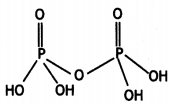(ii)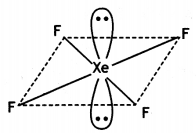OR

(a) Which poisonous gas is evolved when white phosphorus is heated with conc. NaOH solution? Write the chemical equation involved.
Phosphine, PH3
P4 + 3NaOH + 3H2O → 3NaH2PO3 + PH3

(b) Which noble gas has the lowest boiling point?
Helium

(c) Fluorine is a stronger oxidising agent than chlorine. Why?
Fluorine has lower bond dissociation enthalpy of F-F bond than Cl-Cl bond of Cl2 and high enthalpy of hydration because of smaller size of F ion. As a result it has greater tendency to accept electron in solution and is stronger oxidising agent than chlorine.

(d) What happens when H3PO3 is heated?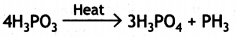(e) Complete the equation:
PbS + O3 → (CBSE 2015)
PbS + 4O3 → PbSO4 + 4O2

Question 30.
(a) Account for the following observations:
(i) SF4 is easily hydrolysed whereas SF6 is not easily hydrolysed.
(ii) Chlorine water is a powerful bleaching agent.
(iii) Bi(V) is a stronger oxidising agent than Sb(V).
(a) (f) S atom in SF4 is not sterically protected as it is surrounded by only four F atoms, so attack of water molecules can take place easily. In contrast, S atom in SF6 is protected by six F atoms. Thus attack by water molecules cannot take place easily.

(ii) Chlorine water produces nascent oxygen (causes oxidation) which is responsible for bleaching action.
Cl2 + H2O → 2HCl + O

(iii) Due to inert pair effect Bi(V) can accept a pair of electrons to form more stable Bi (III) (+3 oxidation state of Bi is more stable than its +5 oxidation state).

(b) What happens when
(i) White phosphorus is heated with concentrated NaOH solution in an inert atmosphere of CO2.
(ii) XeF6 undergoes partial hydrolysis.
(Give the chemical equations involved).
(i) Phosphorus undergoes disproportionation reaction to form phosphine gas.
P4 + 3NaOH + 3H2O → PH3 + 3NaH2PO2

(ii) On partial hydrolysis, XeF6 gives oxyfluoride XeOF4 and HF.
XeF6 + H2O → XeOF4 + 2HF

OR

(a) What inspired N.Bartlett for carrying out reaction between Xe and PtF6?
N. Bartlett first prepared a red compound O2+PtF6. He then realised that the first ionisation enthalpy of molecular oxygen was almost identical with Xenon. So he carried out reaction between Xe and PtF6.

(b) Arrange the following in the order of property indicated against each set:
(i) F2, I2, Br2, Cl2 (increasing bond dissociation enthalpy)
(ii) NH3, ASH3, SbH3, BiH3, PH3 (decreasing base strength)
(i) I2 < F2 < Br2 < Cl2
(ii) NH3 > PH3 > ASH3 > SbH3 > BiH3

(c) Complete the following equations:
(i) Cl2 + NaOH (cold and dilute) →
(ii) Fe3+ + SO2 + H2O →
(CBSE Sample Paper 2018)
(i) 2NaOH + Cl2 → NaCl + NaOCl + H2O
(ii) 2Fe3+ + SO2 + 2H2O → 2Fe2+ + SO42- + 4H+

Question 31.
(a) Give reasons:
(i) H3PO3 undergoes disproportionation reaction but H3PO4 does not.
(ii) When Cl2 reacts with excess of F2, ClF3 is formed and not FCl3.
(iii) Dioxygen is a gas while Sulphur is a solid at room temperature.
(i) In +3 oxidation state phosphorus tends to disproportionate to higher and lower oxidation states / Oxidation state of P in H3PO3 is +3 so it undergoes disproportionation but in H3PO4 it is +5 which is the highest oxidation state.

(ii) F cannot show positive oxidation state as it has highest electronegativity/ Because Fluorine cannot expand its covalency / As Fluorine is a small sized atom, it cannot pack three large sized Cl atoms around it.

(iii) Oxygen has multiple bonding due to pπ-pπ bonding whereas sulphur shows catenation. Oxygen is diatomic therefore held by weak intermolecular force while sulphur is polyatomic and held by strong intermolecular forces.

(b) Draw the structures of the following:
(i) XeF4
(ii) HClO3
(i)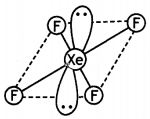(ii)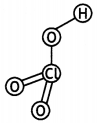OR

(a) When concentrated sulphuric acid was added to an unknown salt present in a test tube a brown gas (A) was evolved. This gas Intensified when copper turnings were added to this test tube. On cooling, the gas (A) changed into a colourless solid (B).
(i) Identify (A) and (B).
(ii) Write the structures of (A) and (B).
(iii) Why does gas (A) change to solid on cooling?
(i) A = NO2, B = N2O4
(ii)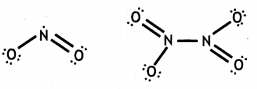(iii) NO2 contains an odd electron. So it dimerises to give N2O4.

(b) Arrange the following In the decreasing order of their reducing character:
HF, HCl, HBr, HI
HI > HBr > HCl > HF

Complete the following reaction:
XeF4 + SbF5 →, (CBSE 2018)
(C) XeF4 + SbF5 → [XeF3] [SbF6]

Question 32.
(i) What happens when
(a) chlorine gas reacts with cold and dilute solution of NaOH?
(b) XeF2 undergoes hydrolysis?
(a) 2NaOH + Cl2 → NaCl + NaOCl + H2O
(cold and dilute)
(b) 2XeF2(s) + 2H2O (l) → 2Xe (g) + 4HF(aq) + O2(S)

(ii) Assign suitable reasons for the following:
(a) SF6 Is inert towards hydrolysis.
(b) H3PO3 is diprotic.
(C) Out of noble gases only Xenon is known to form established chemical compounds.
(a) Sulphur is sterically protected by six F atoms, hence does not allow the water molecules to attack.

(b) It contains only two ionisable H-atoms which are present as -OH groups, thus behaves as dibasic acid.

(c) Xe has least ionisation energy among the noble gases and hence it forms chemical compounds particularly with O2 and F2.

OR

(i) Considering the parameters such as bond dissociation enthalpy, electron gain enthalpy and hydration enthalpy, compare the oxidising power of F2 and Cl2.
F2 is stronger oxidising agent than Cl2. This can be explained on the basis of bond dissociation enthalpy, electron gain enthalpy and hydration enthalpy.
The process of oxidising behaviour may be expressed as:The overall tendency for the change (i.e. oxidising behaviour) depends upon the net effect of three steps. As energy is required to dissociate or convert molecular halogen into atomic halogen, the enthalpy change for this step is positive. On the other hand, energy is released in steps (II) and (III), therefore, enthalpy change for these steps is negative.

Now although fluorine has less negative electron gain enthalpy, yet it is stronger oxidising agent because of low enthalpy of dissociation and very high enthalpy of hydration. In other words, large amount of energy released in step (III) and lesser amount of energy required in step (I) overweigh the smaller energy released in step (II) for fluorine. As a result, the AH overall is more negative for F2 than for Cl2.
Hence, F2 is stronger oxidising agent than Cl2.

(ii) Complete the following reactions :
(a) Cu + HNO3(dilute) →
(b) Fe3+ + SO2 + H2O →
(c) XeF4 + O2F2 → (CBSE 2018)
(a) 3Cu + 8 HNO3 (dilute) → 3Cu(NO3)2 + 2NO + 4H3O
(b) 2Fe3+ + SO3 + 2H3O → 2 Fe2+ + SO42- + 4H+
(c) XeF4 + O2F2 → XeF6 + O2

Question 33.
A crystalline solid ‘A’ burns in air to form a gas ‘B’ which turns lime water milky. The gas is also produced as a by-product during roasting of sulphide ore. This gas decolourises acidified KMnO4 (aq.) solution and reduces Fe3+ to Fe2+. Identify ‘A’ and ‘B’ and write the reactions involved. (CBSE 2019C)
A = S8 / Sulphur
S8 + 8 O2 → 8SO2 / S + O2 → SO2
B = SO2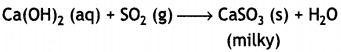Decolourises KMnO4
2KMnO4 + 5 SO2 + 2H4O → 2H2SO4 + 2MnSO4 + K2SO4 / 2MnO4 + 5SO2 + 2H2O → 4H+ + 2Mn2+ + 5SO42-
Reduces Fe3+ to Fe2+
2Fe3+ + SO2 + 2 H2O → 2 Fe2+ + SO42- + 4H+

OR

(a) Arrange the following hydrides of Group 16 elements in the decreasing order of their acidic strength:
H2O, H2S, H2Se, H2Te
H2Te > H2Se > H2S > H2O

(b) Which one of PCl4+ and PCl4 is not likely to exist and why?
PCl4- is not likely to exist because lone pair on P in PCl3 can be donated to Cl+ and not to Cl. Phosphorus has 10e which cannot be accommodated in sp3 orbitals.

(c) Which aliotrope of sulphur is thermally stable at room temperature?